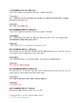# Math: 2nd grade Warm Up ProblemsSubject
Resource Type
File Type

PDF

(376 KB|26 pages)
Product Rating
Standards
• Product Description
• StandardsNEW
In this eBook Timmy tackles the Common Core math standards of 2nd grade by working his way through 54 problems (2 per standard). These are great problems to be used as a warm up or discussed as a class. Follow Timmy's adventures at he has fun in Killington, VT!
Partition circles and rectangles into two, three, or four equal shares, describe the shares using the words halves, thirds, half of, a third of, etc., and describe the whole as two halves, three thirds, four fourths. Recognize that equal shares of identical wholes need not have the same shape.
Partition a rectangle into rows and columns of same-size squares and count to find the total number of them.
Recognize and draw shapes having specified attributes, such as a given number of angles or a given number of equal faces. Identify triangles, quadrilaterals, pentagons, hexagons, and cubes.
Use addition to find the total number of objects arranged in rectangular arrays with up to 5 rows and up to 5 columns; write an equation to express the total as a sum of equal addends.
Determine whether a group of objects (up to 20) has an odd or even number of members, e.g., by pairing objects or counting them by 2s; write an equation to express an even number as a sum of two equal addends.
Total Pages
26 pages
Included
Teaching Duration
N/A
Report this Resource to TpT
Reported resources will be reviewed by our team. Report this resource to let us know if this resource violates TpT’s content guidelines.